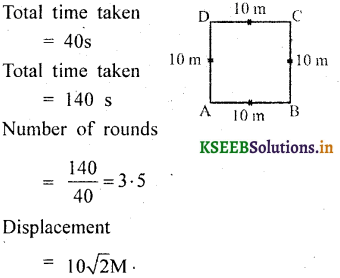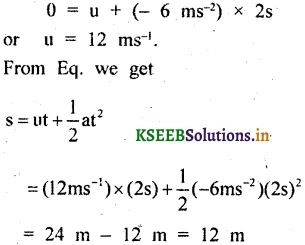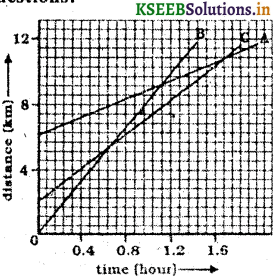# KSEEB Solutions for Class 9 Science Chapter 8 Motion

Students can Download Science Chapter 8 Motion Questions and Answers, Notes Pdf, KSEEB Solutions for Class 9 Science helps you to revise the complete Karnataka State Board Syllabus and to clear all their doubts, score well in final exams.

## Karnataka State Syllabus Class 9 Science Chapter 8 Motion

### KSEEB Class 9 Science Motion Intext Questions and Answers

Question 1.
An object has moved through a distance can it have zero displacements? If yes support your answer with an exonic.
Yes.
If its initial and terminal point remains the same.Point A and Point B, remains the same, but the distance travelled by a body from a point to itself.

Question 2.
A farmer moves along the boundary of a square field of side 10 m in 10 sec. What will be the magnitude of displacement of the farmer at the end of 2 minutes 20 seconds from what position?
Total side × 4 = 10 × 4 = 40 mQuestion 3.
Which of the following is true for displacement?
(a) it cannot be zero
False

(b) its magnitude is greater than the distance travelled by the object
False

Question 4.
Distinguish between speed and velocity.

 Speed Velocity It indicates the rate at which objects move It indicates the rate at which objects move and even the direction of the object.

Question 5.
Under what condit10ns is the magnitude of the average velocity of an object equal to the average speed.
The velocity of an object is uniform or variable. it can be changed by changing the object speed. The described path remains the same. (Average speed)

Question 6.
What does the odometer of an automobile measure?
Distance travelled by an object.

Question 7.
What does the path of an object look like when it is uniform mot10n?
It described equal distances in equal intervals of time.
(Uniform mot10n, speed is constant direct10n may charge)

Question 8.
During an experiment, a signal from a space ship reached the ground stat10n in 5 minutes what was the distance of the spaceship from the ground stat10n? The signal travelled at the ground stat10n? The signal travelled at the speed of light that is 3 × 10 rn/s.
Distance speed × time
= 3 × 108 × 5 × 60
300 × 108 m × 3
9 × 1010 M.

Acceleration calculator is a tool that allows you to calculate acceleration corresponding to different acceleration formulas.

Question 9.
When will you say a body is in (i) uniform accelerat10n (ii) non-uniform accelerat10n?

 Uniform acceleration Non-uniform acceleration If an object travels in a straight line and its velocity increases or decreases by equal amounts in equal intervals of time. Velocity charges with unequal intervals of time if it travels along with a straight line increases if its speed by unequal amounts in equal intervals of time.

Question 10.
A bus decreases its speed from 80 km h-1 to 60 km h-1 in 5 seconds find acceleration of the bus
u = 22.22 m/s
a = 1.11 m/s2 (Negative accelerat10n).

Question 11.
A train starting from a railway stat10n and moving with, uniform accelerat10n attains a speed 40 kmh-1 in 10 minutes find its acceleration.
V = 11.11 mis ;
t = 10 x 60 = 600 s
$$a=\frac{v-u}{t}=\frac{11 \cdot 1-0}{600}$$
=1.85 x 10-2 m/s2
=0.018 m/s2

Question 12.
What is (the nature of the distance-time graph for uniform and non-uniform mot10n of an object?
(i) Uniform motion: Straight line and parallel to the x-axis. (strongest line under saine scope)
(ii) Non-uniform motion: Magnitude of displacement equal to the curved hue.

Question 13.
What can you say about the mot10n (of an object whose distance-time graph ¡s a straight line parallel to the time axis
Uniform velocity (Object’s stationery)

Question 14.
What can OU say about the mot10n of in object if its speed-time graph is a straight line parallel to the time axis.
No. Accelerat10n :

Question 15.
What ¡s the quantity which is mçasurcd by the area occupied below the velocity-time graph.
Distance travelled by displacement.

Question 16.
A bus starting from rest moves with uniform accelerat10n of 0.1 mins for 2 minutes. Find (a) the speed acquired (b) the distance travelled.
(a) u = 0, a 0.1 rns-2,
t = 2 min 2 x 60 = l20 sec
V = u + at
V = 0 + 0. 1 x 120 = 12 m/s.

(b) $$\mathrm{S}=\mathrm{ut}+\frac{1}{2} \mathrm{at}^{2}$$
\begin{aligned} &=0 \times 120+\frac{1}{2} \times 0 \cdot 1 \times(20)^{2}\\ &=7 \cdot 2 \times 10^{2} \mathrm{M}=720 \mathrm{M} \end{aligned}

Question 17.
A train is travelling at a speed of 90 km lr1 brakes are applied so as to produce a uniform accelerat10n of – 0.5 ms-2 find how far the train will go before it is brought to rest.
S = ? u= 0, v – 90 knih
a = – 0.5 ms-2
V2 = u2 + 2as
$$S=\frac{v^{2}-u^{2}}{2 a s}=\frac{0-(25)^{2}}{2 \times(-0 \cdot 5)}=625 \mathrm{M}$$

Question 18.
A trolley, while going down an inclined plane has an accelerat10n of 2 ms 2 what will be its velocity 3 sec after the start.
V = u + at = 0 + 2 x 3 = 6m/s

Question 19.
A racing car has a uniform accelerat10n of 4 ms 2 what distance will it cover in IQs after the start.
\begin{aligned} S &=u t+\frac{1}{2} a t^{2} \\ &=(0 \times 10)+\left(\frac{1}{2} \times 4 \times 10^{2}\right)=200 \mathrm{M} \end{aligned}

Question 20.
A stone is thrown in a vertically upward direct10n with a velocity of 5 ms-1 of the accelerat10n of the stone Differentiating its mot10n is 10 ms-2 in the downward direct10n what will be the height attained by the stone and how much time will it make to reach there?
V2 = u2 + 2as
$$\ S=\frac{v^{2}-u^{2}}{2 a}=\frac{0 \cdot(5)^{2}}{2 \times(-10)}=1 \cdot 25 \mathrm{M}$$
\begin{aligned} &\mathrm{V}=\mathrm{u}+\mathrm{at}\\ &t=\frac{v-u}{a}=\frac{0-5}{-10}=0 \cdot 5 \mathrm{sec} \end{aligned}

Question 21.
A car accelerates uniformly from 8 km h-1 to 36 km or’ in 5 s. Calculate (i) the accelerat10n and (ii) the distance covered by the car in that time.
We are given thatThe accelerat10n of the car is 1 in ms-2 and me distance covered is 37.5 m.

Question 22.
The brakes applied to a car produce an accelerat10n of 6 m s-2s in the opposite direct10n to the mot10n. If the car takes 2 s to stop after the applicat10n of brakes, calculate the distance it travels during this time.
We have been given
a = – 6 ms-2; t = 2 s and v = 0 ms-1
From Eq. we know that
v = u + at.Thus, the car will move 12 m before it stops after the applicat10n of brakes. Can you now appreciate why drivers are caut10ned to maintain some distance between vehicles while travelling on the road? ,

### KSEEB Class 9 Science Motion Textbook Exercise Questions and Answers

Question 1.
An athlete completes one round of a circular track of diameter 200 m • in 40 s. What will be the distance covered and displacement at the end of 2 minutes 20 s?
\begin{aligned} 2 \pi r=\frac{22}{7} \times 200 &=628 \cdot 57 \mathrm{M} \\ &=\frac{628 \cdot 57}{40}=15 \cdot 7 \mathrm{m} \end{aligned}

Question 2.
Joseph jogs from one end A to the other end B of a straight 300 m road in 2 minutes 30 seconds and then turns around and jogs 100 m back to point C in another 1 minute. What Joseph’s average speeds and velocities in jogging (a) from A and B and (b) from A to C?
A to B = 150 sec.Question 3.
Abdul, while driving to school, computes the average speed for his trip to be 20 km h~‘ On his return trip along the same route, there is less traffic and the average speed 30 km h1. What is the average speed for Abdul’s trip?
Average, speed 20 km/h
Total distance = 2x
Average speed = $$\frac{2 x \times 60}{5 x}=24 k m / h$$

Question 4.
A motorboat starting from rest on a lake accelerates in a straight line at a constant rate of 3.0 ms-2 for 8.0 s. How far does the boat travel during this time?
V2 = 2as ;
S = ut + $$\frac{1}{2}$$at2
S = $$\frac{1}{2}$$ x 3 x 64 = 96M

Question 5.
A driver of a car travelling at 52 km h-1 applies the brakes and accelerates uniformly in the opposite direct10n. The car stopped in 5 s. Another driver going at 3 km h-1 in another car applies his brakes slowly and stops in 10 s. On the same graph paper plot the speed versus time graphs for the two cars. Which the two cars travelled farther after the brakes were applied?
Initial speed = $$\frac{52 \times 100}{60 \times 60}$$
1st driver = 14.44 m/s.
2nd driver = $$\frac{3 \times 1000}{60 \times 60}=0.833 \mathrm{cm}$$

Question 6.
Fig. shows the distance-time graph of three objects A, B and C. Study the graph and answer the following questions:(a) Which of the three is travelling the fastest?
(b) Are all three ever at the same point on the road?
(c) How far has C travelled when B passes A?
(d) How far has B travelled by the time it passes C?
(a) B is travelling fastest.
(b) No. three lines do not intersect at any point.
(c) When B. passes A. C is at a distance approximately 8 km from the origin.
(d) By the time B. passes C it has travelled 5.5 km,

Question 7.
A ball is gently dropped from a height of 20 m. If its velocity increases uniformly at the rate of 10 m s-2, with what velocity will it strike the ground? After what time will it strike the ground?Question 8.
The speed-time graph for a car is shown is Fig.(a) Find how far does the car travel in the first 4 seconds. Shade the area on the graph that represents the distance travelled by car during the per10d.
(b) Which part of the graph represents the uniform mot10n of the car?
(a) Distance travelled by car in the first 4 seconds nearly 12 m.
(b) Uniform mot10n of the car is shown after 5.5 sec.

Question 9.
State which of the following situat10ns are possible and give an example for each of these:
(a) an object with a constant accelerat10n but with zero velocity.
(b) an object moving in a certain direct10n with an accelerat10n in the perpendicular direct10n.
(a) Influencing of gravity.
(b) the object has a circular path in mot10n.

Question 10.
An artificial satellite is moving in a circular orbit of radius 42250 km. Calculate its speed if it takes 24 hours to revolve around the earth.
= 42250 x 1000 M
Time required = 24 hours
= 24 x 3600 sec
Speed = ?
\begin{aligned} \text { Speed } &=\frac{\text { distance }}{\text { time }}=\frac{2 \pi r}{\text { time }} \\ &=2 \times \frac{22}{7} \times \frac{42250 \times 1000}{24 \times 3600} \end{aligned}
Speed = 3073.74 m/s

Question 1.
Give an express10n for centripetal force?
$$f=\frac{m v^{2}}{r}$$

Question 2.
Define inertia.
The tendency of a body to remain hr the state of rest or uniform mot10n.

Question 3.
What is uniform circular mot10n?
Body moving in a circular path arbitrary any instant along a tangential to the posit10n of the body on the circular path at that instant or time.

Question 4.
Is the mot10n of moon around the earth uniform or accelerated?
Mot10n of the moon around the earth is an accelerated mot10n due to continuous charge in the direct10n of mot10n.

Question 5.
A body falls freely. What is constant?
When the body falls freely. It has constant acceleration.

Question 6.
Can distance be zero, even when the displacement is not zero?
Average speed = $$\frac{u+v}{2}$$